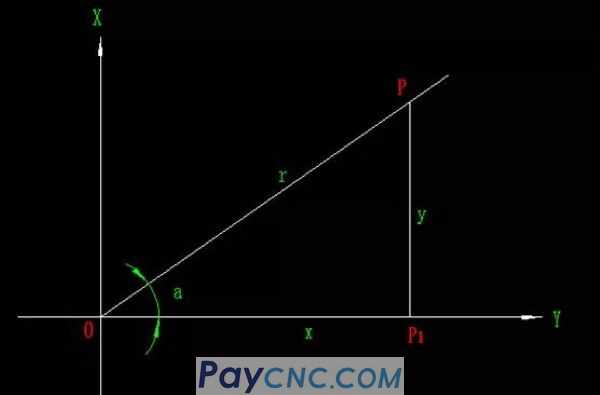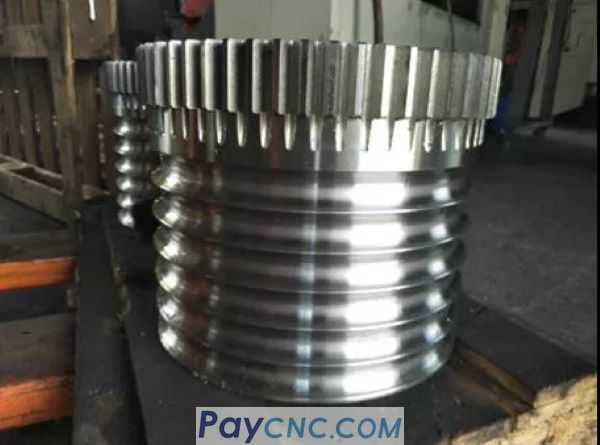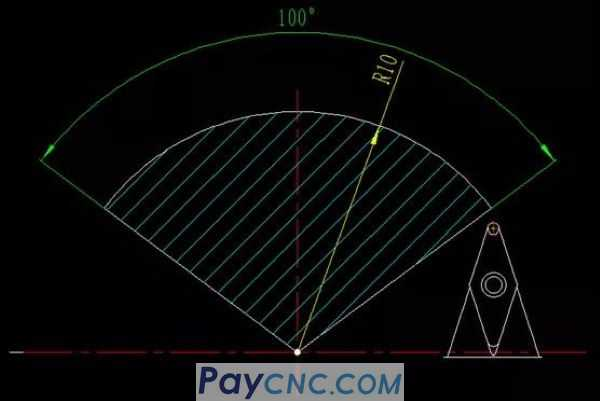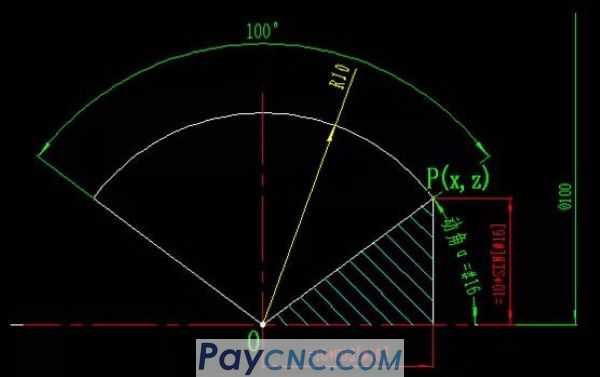After learning the macro program, it is very convenient and not difficult to apply. Apply a formula to find the coordinate points according to the variables..."   Today I share an example of trigonometric function application that is frequently used   1. Trigonometric function formula   2. Trigonometric function application case   One, the trigonometric function formula   In the right triangle OPP1, the ratio between the opposite side y of the angle a, the adjacent side x and the hypotenuse r defines the trigonometric function of the angle a. There are three commonly used mathematical formulas as follows:sin a=y/r cos a=x/r tan a=y/x   Many friends will ask how these trigonometric formulas come from. In fact, you don’t need to know how the formulas come from. This is a matter of studying mathematics (which should have been covered in mathematics textbooks). We only need to figure out the relationship between the above formulas. .   That is: knowing two data, the other data can be calculated by the above formula   For example, given the degree of the included angle a and the length of side r, how to calculate the side length of x and y?   According to the formula: cos a=x/r can be calculated x=r* cos a   According to the formula: sin a=y/r, y=r* sin a can be calculated   Two, trigonometric function application case   For example, such as the lower arc threadThe following diagram: Machining a circular arc thread with a radius of R10 and a radian of 100 degrees.How to calculate the point coordinates on the arc when machining the arc thread?   This involves trigonometric functions, as shown in the following diagram:P is any point on the arc, and #16 is the dynamic angle between point P and X axis, calculated according to the trigonometric formula:   Z coordinate on point P: 10*COS[#16];   X coordinate on point P: 10*SIN[#16];   In this way, with the automatic increment operation of the dynamic included angle #16 (how to understand the automatic increment operation of variables, please refer to the link at the end of the article), the point coordinates on the arc are calculated by trigonometric functions;   The procedure is as follows: